[BACK]
 Computer Systems Science & EngineeringDOI:10.32604/csse.2023.026011Article

Combining Entropy Optimization and Sobel Operator for Medical Image Fusion

1Faculty of Computer Science and Engineering, Thuyloi University, 175 Tay Son, Dong Da, Hanoi, 010000, Vietnam
2University of Information and Communication Technology, Thai Nguyen University, Thai Nguyen, 240000, Vietnam
*Corresponding Author: Nguyen Tu Trung. Email: trungnt@tlu.edu.vn
Received: 13 December 2021; Accepted: 14 January 2022

Abstract: Fusing medical images is a topic of interest in processing medical images. This is achieved to through fusing information from multimodality images for the purpose of increasing the clinical diagnosis accuracy. This fusion aims to improve the image quality and preserve the specific features. The methods of medical image fusion generally use knowledge in many different fields such as clinical medicine, computer vision, digital imaging, machine learning, pattern recognition to fuse different medical images. There are two main approaches in fusing image, including spatial domain approach and transform domain approachs. This paper proposes a new algorithm to fusion multimodal images. This algorithm is based on Entropy optimization and the Sobel operator. Wavelet transform is used to split the input images into components over the low and high frequency domains. Then, two fusion rules are used for obtaining the fusing images. The first rule, based on the Sobel operator, is used for high frequency components. The second rule, based on Entropy optimization by using Particle Swarm Optimization (PSO) algorithm, is used for low frequency components. Proposed algorithm is implemented on the images related to central nervous system diseases. The experimental results of the paper show that the proposed algorithm is better than some recent methods in term of brightness level, the contrast, the entropy, the gradient and visual information fidelity for fusion (VIFF), Feature Mutual Information (FMI) indices.

Keywords: Medical image fusion; wavelet; entropy optimization; PSO; Sobel operator

1  Introduction

Fusing medical images is combining the information of multimodality images to acquire accurate information . This fusion aims to improve the image quality and preserve the specific features. An overview of the techniques of image fusion applied into medical applications can be seen in . The methods of medical image fusion generally use knowledge in many different fields such as clinical medicine, computer vision, digital imaging, machine learning, pattern recognition to fuse different medical images .

There are two main approaches in fusing image, including spatial domain approach and transform domain approachs . With the spatial domain approach, the fused image is chosen from the regions/pixels of the input images without transformation . This approach includes the region based  and pixel based  methods. The techniques of transform domain do fusing the corresponding transforming coefficients and later apply the inverse transformation for producing the fused image. One of the popular fusion techniques is transform of multi scales. There are various multi transform based on contour transform , a complex wavelet transform , the discrete wavelet transform  or sparse representing .

Recently, there are many new techniques in fusing images. Mishra et al.  presented a method of fusing Computed Tomography-Magnetic Resonance Imaging (CT-MRI) images using discrete wavelet transform. In  and , the authors introduced a method of fusing images using the Principal Component Analysis (PCA). Sarmad et al. proposed a method of fusing multimodal medical images by applying sparse representing and two-scale decomposing techniques on images . Xu et al.  proposed a method of fusing medical images using hybrid of wavelet-homomorphic filter and an algorithm of modified shark smell optimization. Polinati et al.  introduced a method of fusing the information of the various image modalities such as speculation (SPEC), positron emission tomography (PET) and MRI using fusion rule of local energy maxima and empirical wavelet transform representation. Hu et al.  presented a fusing method of combining dictionary optimization and the filter Gabor in contourlet transform domain. Chen et al.  proposed a method of medical image fusion that is based on Rolling Guidance Filtering. Haribabu et al.  showed statical measurements of fusing medical images for MRI-PET images using 2D Herley transform with HSV color space. Manchanda et al.  improved an algorithm of medical image fusion by using fuzzy transformation (FTR). In , a new algorithm for fusing medical images was proposed. This algorithm used lifting scheme based bio-orthogonal wavelet transform. Hikmat Ullah et al. proposed a method of fusing multimodality medical images. This method is based on fuzzy sets with local features and new sum-modified-Laplacian in domain of the shearlet transform . In , Liu et al. introduced a new method of fusing medical images that is Convolutional Sparsity-based by Analysis of Morphological Component.

The new techniques which are based deeplearning, are proposed recently. In , a medical image fusion method based on convolutional neural networks (CNNs) is proposed. In our method, a siamese convolutional network is adopted to generate a weight map which integrates the pixel activity information from two source images. B. Yang et al.  present a novel joint multi-focus image fusion and super-resolution method via convolutional neural network (CNN). While a novel jointed image fusion and super-resolution algorithm is proposed in . And Jiayi Ma et al. proposed a new end-to-end model, termed as dualdiscriminator conditional generative adversarial network (DDcGAN), for fusing infrared and visible images of different resolutions .

The medical image fusion approach, uses wavelet transform, usually applies the average selection rule on low frequency components and max selection rule on high frequency components. This causes the resulting image to be greatly grayed out compared to the original image because the grayscale values of the frequency components of the input images differ greatly. In addition, some recent methods focus mainly on the fusion so that they can reduce the contrast and brightness of the fused image. This makes it difficult to diagnose and analyze based on the fused image. To overcome the limitations, this paper proposes a novel algorithm for fusing multimodal images by combining of Entropy optimization and the Sobel operator.

•   Propose a new algorithm based on the Sobel operator for combining high frequency components.

•   Propose a novel algorithm that is used for fusing multimodal images based on wavelet transform.

•   Propose a new algorithm based on the Sobel operator for combining low frequency components. This algorithm is combined by Entropy based on parameter optimization using PSO algorithm. The fusion image preserves colors and textures similarly to input image.

The remaining of this article is structured as follows. In Section 2, some related works are presented. The proposed algorithm about image fusion is presented in Section 3. Section 4 presents some experiments of our algorithm and other related algorithms on selected images. Conclusions and the future researches are given in Section 5.

2  Background

2.1 Wavelet Transformation

Wavelet Transformation (WT) is a mathematical tool . This tool is used for presenting images with multi-resolution. After transforming, wavelet coefficients is obtained. For remote sensing images, wavelet coefficients can be obtained by Discrete Wavelet Transform (DWT). In which, the most important content is low frequency. This content keeps most of the features of input image and its size is decreased by four times. By using low pass filter with two directions, the approximate image (LL) is achieved.

When DWT performed, the size of image LL is four times smaller than the image LL of the previous stage. Therefore, if the input image is disaggregated into 3 levels, size of the final approximate image is 64 times smaller than the input image. Wavelet transformation of image is illustrated as in Fig. 1.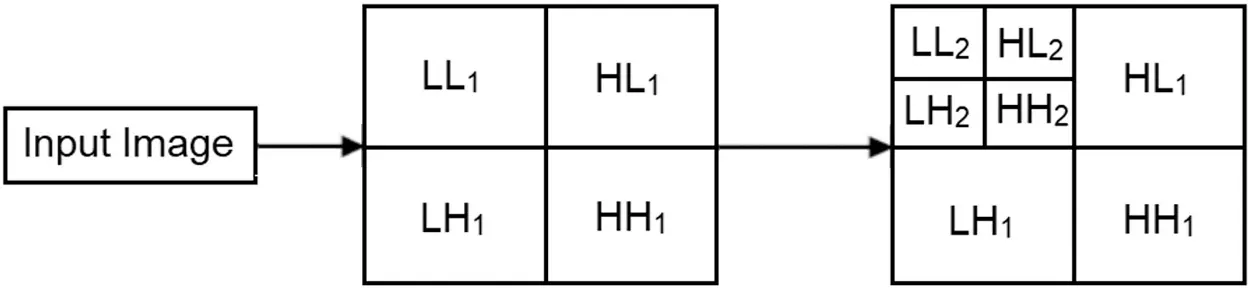Figure 1: Image Decomposition using DWT

2.2 Particle Swarm Optimization (PSO)

PSO is an algorithm about finding solutions to optimization problems . This is the result of modeling bird flocks that fly to find foods. In many fields, this algorithm was successfully applied. First, PSO initialized a group of individuals randomly. Then, the algorithm updated generations to find the optimal solution. With each generation, two best positions of each individual was updated, denoted as PI_best and GI_best. Wherein the first value, PI_best is best the position that has ever reached. GI_best is the best position that obtained in the whole search process of the population up to the present time. Specifically, after each generation was updated, velocity and the position of each individual are updated by following formulas:

XIik+1=XIik+VIik+1 (1)

VIik+1=ω×VIik+c1×r1×(PIbestikXIik)+c2×r2×(GIbestkXIik) (2)

where:

•    XIik: Position of the individual ith in generation kth.

•    VIik: Velocity of the individual ith in generation kth.

•    XIik+1: Position of the individual ith in generation (k+1)th.

•    VIik+1: Velocity of the individual ith in generation (k+1)th.

•    PIbest_ik: Best position of the individual ith in generation kth.

•    GIbestk: Best position of in population in generation kth.

•    ω = 0.729 is the inertia coefficient.

•    c1 , c2 : The acceleration coefficients, getting values from 1.5 to 2.5.

•    r1 , r2 : Random numbers get values in the range [0,1].

2.3 Fusing Images Based on Wavelet Transformation

Reference  presented a method of fusing CT-MRI images based on the discrete wavelet transform (WIF), as shown in Fig. 2.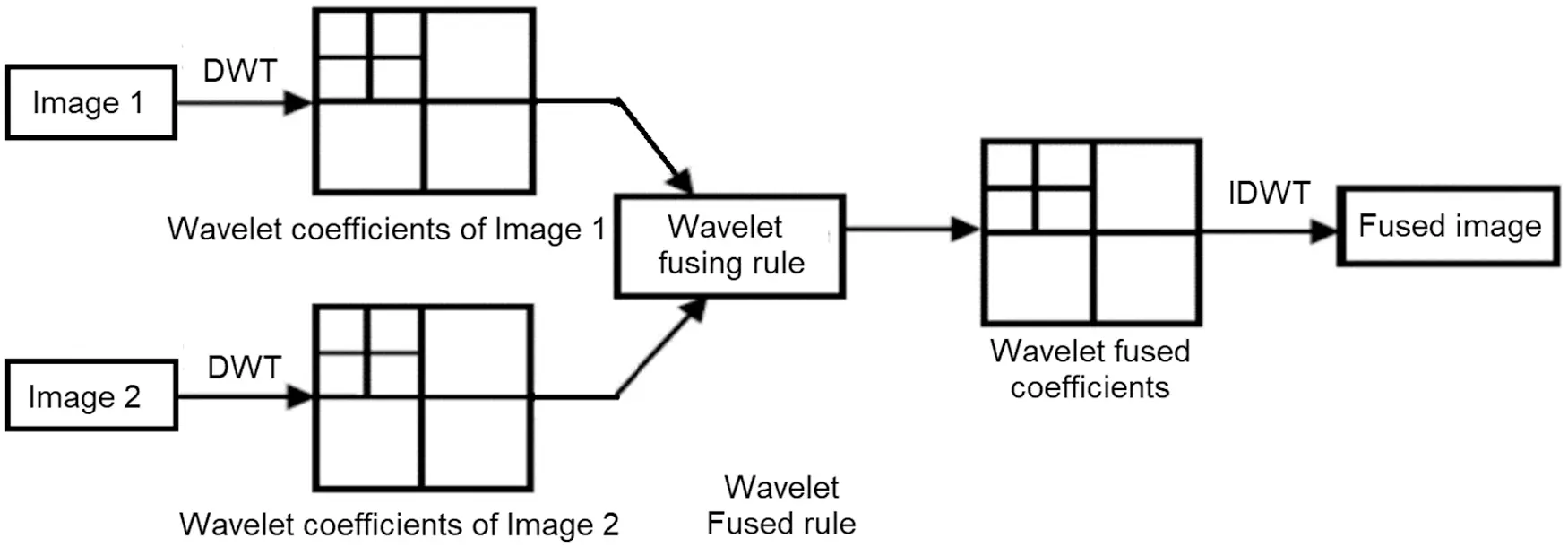Figure 2: The chart of fusing image using the wavelet transform

With IA(xp,yp) , IB(xp,yp) are two input images and IF(xp, yp) is fused image, fusion rule includes:

•   Average method:

IF(xp,yp)=(IA(xp,yp)+IB(xp,yp))/2 (3)

•   Select Maximum:

IF(xp,yp)=Max(IA(xp,yp),IB(xp,yp)) (4)

•   Select Minimum:

F(xp,yp)=Min(IA(xp,yp),IB(xp,yp)) (5)

3  The Proposed Method

3.1 The Algorithm of Combining High Frequency Components Based on Sobel Operator

The algorithm of combining high frequency components based on Sobel operator (CHCSO) is stated as follows:

Input: Two high frequency components H1 , H2 .

Output: Combining component.

The main steps of CHCSO include:

Step 1: Get H1S edge component of H1 with Sobel operator.

Step 2: Get H2S edge component of H2 with Sobel operator.

Step 3: Combine component HF as below:

HF(xp,yp)={H1(xp,yp)if|H1S(xp,yp)||H2S(xp,yp)|H2(xp,yp)if|H1S(xp,yp)||H2S(xp,yp)| (6)

3.2 The Medical Image Fusion Algorithm

In this section, a new algorithm for fusing medical images named as the Entropy optimization and Sobel operator based Image Fusion (ESIF) is proposed. The general framework of the algorithm ESIF is shown in Fig. 3 below.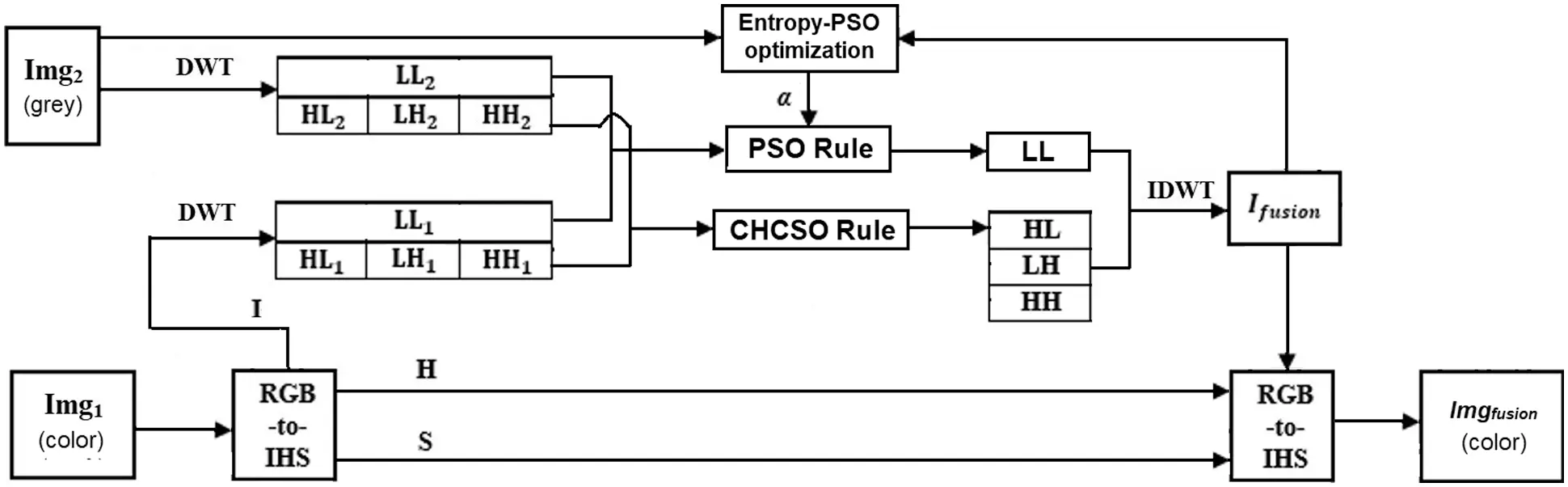Figure 3: The framework of the algorithm of medical image fusion ESIF

Where, Img1 is PET or SPEC image (color images), Img2 is CT or MRI image (grey images).

According to Fig. 3, the algorithm includes the following steps:

•   Step 1: Convert image img1 in Red, Blue and Green (RGB) color space to Hue, Saturation, Intensity (HIS) color space to get IImg1, HImg1, SImg1.

•   Step 2: Transform IImg1 and IImg2 to get HL1, LL1, HH1, LH1 and HL2, LL2, HH2, LH2 using DWT transformation.

•   Step 3: Fuse the high frequency components (HL1, LH1, HH1) and (HL2, LH2, HH2) to get HL, LH, HH using the rule which is based on the algorithm CHCSO as follows:

LH=CHCSO(LH1,LH2) (7)

HL=CHCSO(HL1,HL2) (8)

HL=CHCSO(HL1,HL2) (9)

•   Step 4: Fuse the low frequency components (LL1) and (LL2) to get LL using the rule as follows:

LL=αLL1+(1α)LL2(10)

The parameter α is found by using an algorithm PSO with the optimization of objective function as follows:

•   Step 5: Transform the components (LL, LH, HL, HH) to get Ifusion using IDWT transformation.

f=(HIfusionHImg2)2 (11)

where, HIfusion is entropy of Ifusion and HImg2 is entropy of Img2.

•   Step 6: Convert the components Ifusion, HImg1, SImg1 in HIS color space to RGB color space to obtain the output fused image.

The proposed algorithm has some advantages, including:

i)  Combining the high frequency components is adaptive using the algorithm CHCSO with the Sobel operator instead of the rule Select Maximum .

ii)  Combining the low frequency components using weighted parameters which are found by using an algorithm PSO with the optimization of objective function in formula (11).

iii)  Overcome the limitations of the approach that is based on wavelet transform as mentioned in section I.

4  Experimental Results

4.1 Experimental Setting

Input data is downloaded from Atlas  with 1500 image files as slices. The image size is 256 × 256. This dataset is used to introduce to basic neuroanatomy, with emphasizing pathoanatomy of some diseases about central nervous system. It includes many different types of medical images such as MRI, PET or SPECT. On this dataset, our proposed algorithm (ESIF) is compared with other available methods, including Wavelet based image fusion (WIF) , PCA based image fusion (PCAIF)  and morphological component analysis based on convolutional sparsity (CSMCA) .

To assess image quality, we use the measures such as the brightness level ( μ ), the contrast ( σ2 ), the entropy (E), the gradient (G), VIFF  and FMI .

4.2 Evaluation Results

Herein, we illustrate the experiment with 5 slices 070, 080 and 090, 004, 007 as below. Input and output images of the fused methods are presented in Tab. 1.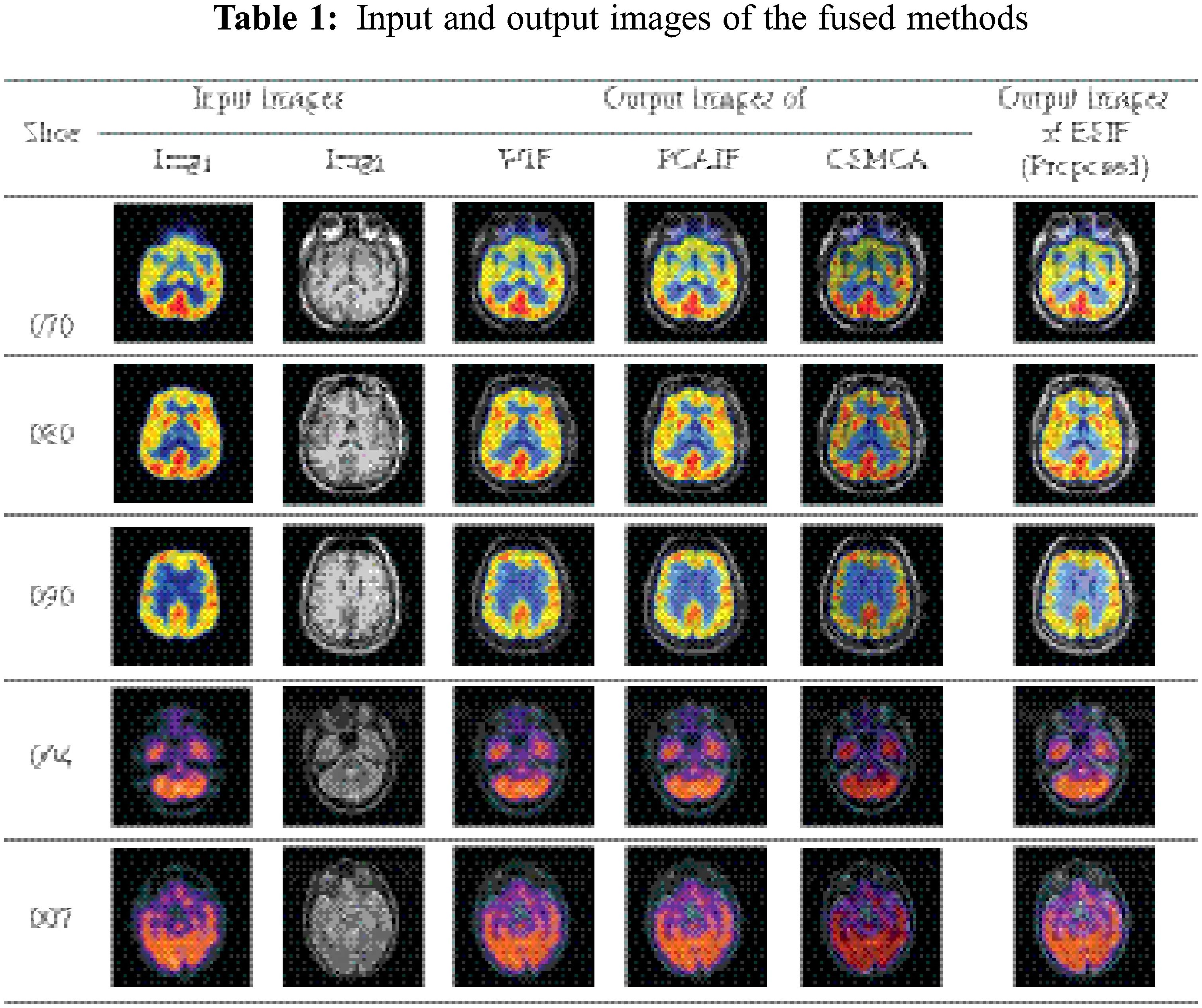From the output images of four methods in Tab. 1, some characteristics of the results can be summarized as below:

•   The WIF and PCAIF methods do not highlight the boundary of the areas in the resulting images.

•   The CSMCA method even generates very dark fused image compared to WIF and PCAIF methods. This makes it difficult to distinguish areas in the image.

•   The fused images generated by the proposed method has better contrast and bright and clearly distinguishing the areas than fused images using the compared methods.

For the quantity evaluation, the values of criteria μ, σ2, E, G, VIFF and FMI indexes of the output images that generated by the fusion methods are calculated and given in Tab. 2 below.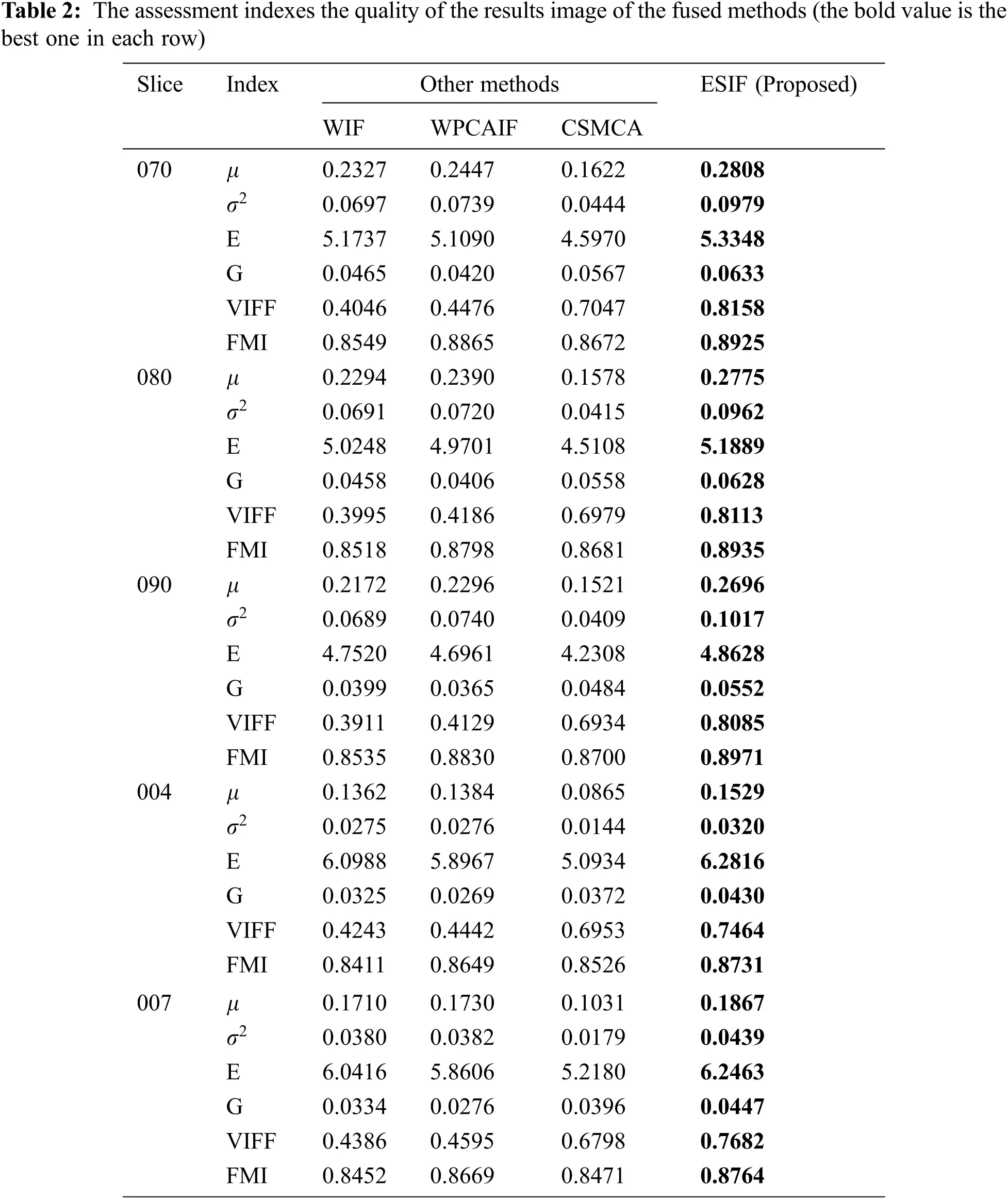From the results in Tab. 2, by using our proposed method, the results of μ , σ2, E, G, VIFF and FMI obtained are the best values on all slices. To compare the results on each criterion, the average values of μ , σ2, E, G, VIFF and FMI indexes obtained by applying four methods on five slices are visually presented as in Fig. 4.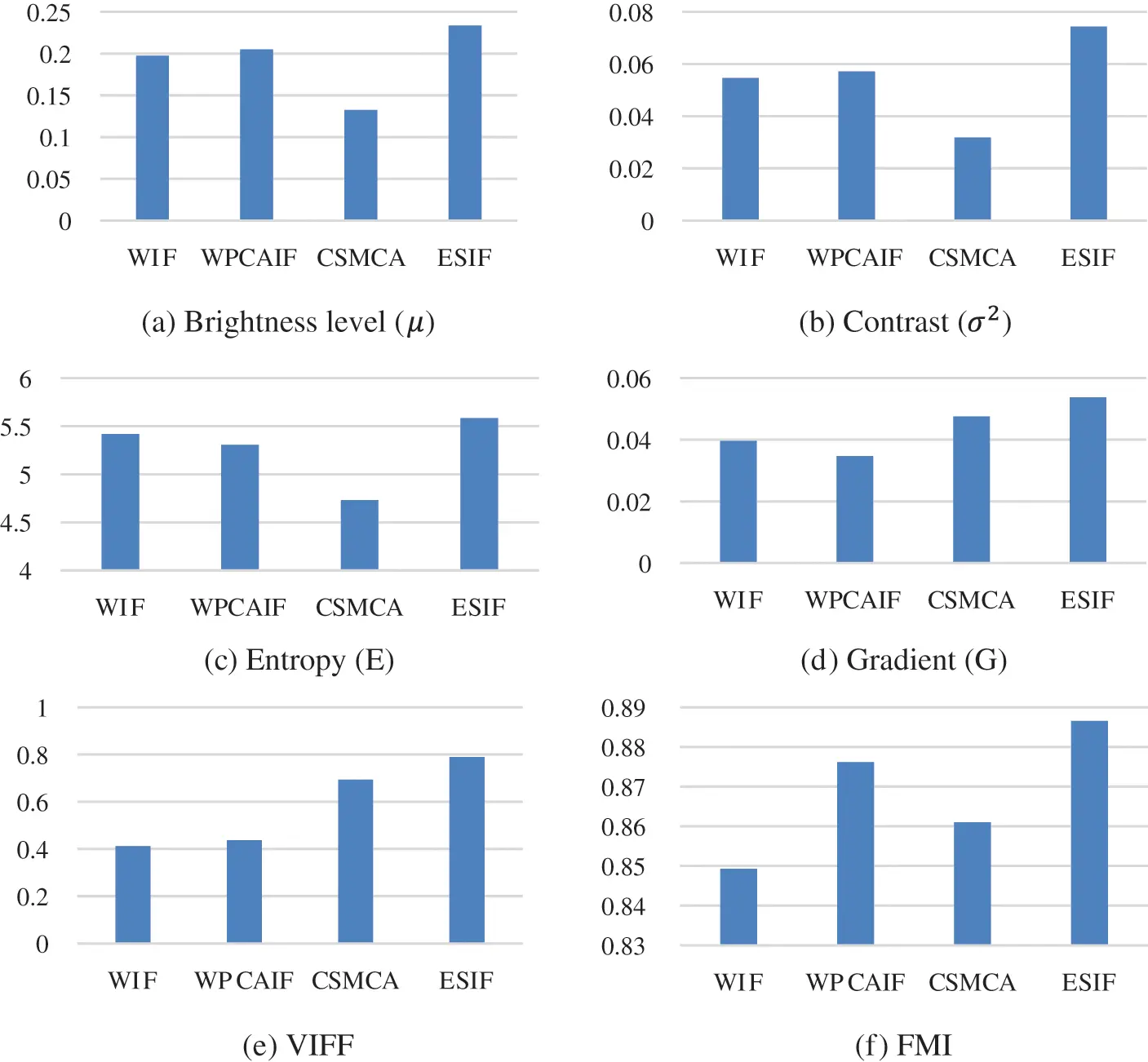Figure 4: Comparison among four methods by the average values on 5 slices of 6 evaluation indices. (a) Brightness level ( μ ) (b) Contrast ( σ2 ) (c) Entropy (E) (d) Gradient (G) (e) VIFF (f) FMI

Fig. 4 shows that the average values of μ , σ2, E obtained by CSMCA are the worst values comparing with those of other methods. However, the average values of G, VIFF and FMI obtained by this method are higher than those of VIF. Comparing with PCAIF, CSMCA is better in two criteria (G and VIFF). This means that the quality of the fused images of the CSMCA method is not always good and unstable.

Moreover, from the results in Tab. 2 and Fig. 4, the values of all criteria achieved by using ESIF are higher than other methods. Especially, the values of ESIF are 1.76 times higher than CSMCA on brightness level; 2.34 times higher than CSMCA on the contrast; 1.92 times higher than VIF on FMI. This leads to conclude that the quality of the fused images when applying our proposed method is much better than three mentioned methods on the same data.

5  Conclusions and Future Works

This paper introduces the new algorithm of fusing multimodal images based on Entropy optimization and the Sobel operator (ESIF). This algorithm aims to get the fused images without reducing the brightness and contrast. The proposed method has advantages as the adaptability of combining the high frequency components by using the algorithm CHCSO with the Sobel operator; the high performance in combining the low frequency components based on the weighted parameter obtained by using an algorithm PSO. Apart from that, our proposed method overcomes the limitations of wavelet transform based approaches.

The experimental results on five different slices of images show the higher performance of proposed method in term the brightness level, the contrast, the entropy, the gradient and VIFF, FMI indices. For further works, we intend to integrate the parameter optimization in image processing and apply the improvement method in other problems.

Funding Statement: The authors received no specific funding for this study.

Conflicts of Interest: The authors declare that they have no conflicts of interest to report regarding the present study.

## References

1. H. Li, Z. Yu and C. Mao, “Fractional differential and variational method for image fusion and super-resolution,” Neurocomputing, vol. 171, no. 9, pp. 138–148, 2016.
2. J. Du, W. Li, K. Lu and B. Xiao, “An overview of multi-modal medical image fusion,” Neurocomputing, vol. 215, no. 4, pp. 3–20, 2016.
3. A. P. James and B. V. Dasarathy, “Medical image fusion: A survey of the state of the art,” Information Fusion, vol. 19, no. 3, pp. 4–19, 2014.
4. S. Li, X. Kang, L. Fang, J. Hu and H. Yin, “Pixel-level image fusion: A survey of the state of the art,” Information Fusion, vol. 33, no. 6583, pp. 100–112, 2017.
5. H. Li, H. Qiu, Z. Yu and B. Li, “Multifocus image fusion via fixed window technique of multiscale images and non-local means filtering,” Signal Processing, vol. 138, no. 3, pp. 71–85, 2017.
6. M. Zribi, “Non-parametric and region-based image fusion with Bootstrap sampling,” Information Fusion, vol. 11, no. 2, pp. 85–94, 2010.
7. S. Yang, M. Wang, L. Jiao, R. Wu and Z. Wang, “Image fusion based on a new contourlet packet,” Information Fusion, vol. 11, no. 2, pp. 78–84, 2010.
8. F. Nencini, A. Garzelli, S. Baronti and L. Alparone, “Remote sensing image fusion using the curvelet transform,” Information Fusion, vol. 8, no. 2, pp. 143–156, 2007.
9. H. Li, H. Qiu, Z. Yu and Y. Zhang, “Infrared and visible image fusion scheme based on NSCT and low-level visual features,” Infrared Physics & Technology, vol. 76, no. 8, pp. 174–184, 2016.
10. B. Yu, B. Jia, L. Ding, Z. Cai, Q. Wu et al., “Hybrid dual-tree complex wavelet transform and support vector machine for digital multi-focus image fusion,” Neurocomputing, vol. 182, no. 11, pp. 1–9, 2016.
11. Y. Yang, “A novel DWT based multi-focus image fusion method,” Procedia Engineering, vol. 24, pp. 177–181, 20
12. B. Yang and S. Li, “Multifocus image fusion and restoration with sparse representation,” IEEE Transactions on Instrumentation and Measurement, vol. 59, no. 4, pp. 884–892, 2009.
13. H. O. S. Mishra and S. Bhatnagar, “MRI and CT image fusion based on wavelet transform,” International Journal of Information and Computation Technology, vol. 4, no. 1, pp. 47–52, 2014.
14. S. Mane and S. D. Sawant, “Image fusion of CT/MRI using DWT, PCA methods and analog DSP processor,” International Journal of Engineering Research and Applications, vol. 4, no. 2, pp. 557–563, 20
15. S. Deb, S. Chakraborty and T. Bhattacharjee, “Application of image fusion for enhancing the quality of an image,” CS & IT, vol. 6, pp. 215–221, 2012.
16. S. Maqsood and U. Javed, “Multi-modal medical image fusion based on two-scale image decomposition and sparse representation,” Biomedical Signal Processing and Control, vol. 57, no. 2, pp. 101810–101817, 2020.
17. L. Xu, Y. Si, S. Jiang, Y. Sun and H. Ebrahimian, “Medical image fusion using a modified shark smell optimization algorithm and hybrid wavelet-homomorphic filter,” Biomedical Signal Processing and Control, vol. 59, no. 4, pp. 101885–101894, 2020.
18. S. Polinati and R. Dhuli, “Multimodal medical image fusion using empirical wavelet decomposition and local energy maxima,” Optik, vol. 205, no. 3, pp. 163947–163979, 2020.
19. Q. Hu, S. Hu and F. Zhang, “Multi-modality medical image fusion based on separable dictionary learning and Gabor filtering,” Signal Processing: Image Communication, vol. 83, pp. 115758–115787, 2020.
20. J. Chen, L. Zhang, L. Lu, Q. Li, M. Hu et al., “A novel medical image fusion method based on Rolling Guidance Filtering,” Internet of Things, vol. 14, no. 3, pp. 100172–100188, 2021.
21. M. Haribabu and V. Guruvaiah, “Statistical measurements of multi modal MRI-PET medical image fusion using 2D-HT in HSV color space,” Procedia Computer Science, vol. 165, no. 38, pp. 209–215, 2019.
22. M. Manchanda and R. Sharma, “An improved multimodal medical image fusion algorithm based on fuzzy transform,” Journal of Visual Communication and Image Representation, vol. 51, no. 2, pp. 76–94, 2018.
23. O. Prakash, C. M. Park, A. Khare, M. Jeon and J. Gwak, “Multiscale fusion of multimodal medical images using lifting scheme based biorthogonal wavelet transform,” Optik, vol. 182, pp. 995–1014, 2019.
24. H. Ullah, B. Ullah, L. Wu, F. Y. Abdalla, G. Ren et al., “Multi-modality medical images fusion based on local-features fuzzy sets and novel sum-modified-Laplacian in non-subsampled shearlet transform domain,” Biomedical Signal Processing and Control, vol. 57, pp. 101724–101738, 2020.
25. Y. Liu, X. Chen, R. K. Ward and Z. J. Wang, “Medical image fusion via convolutional sparsity based morphological component analysis,” IEEE Signal Processing Letters, vol. 26, no. 3, pp. 485–489, 2019.
26. Y. Liu, X. Chen, J. Cheng and H. Peng, “A medical image fusion method based on convolutional neural networks,” in Proc. of 20th Int. Conf. on Information Fusion, pp. 1–7, 2017.
27. B. Yang, J. Zhong, Y. Li and Z. Chen, “Multi-focus image fusion and superresolutionwith convolutional neural network,” in Int. J. Wavelets Multiresolut. Inf. Process, vol. 15, no. 4, pp. 1–15, 2017.
28. J. Zhong, B. Yang, Y. Li, F. Zhong and Z. Chen, “Image fusion and super-resolution withconvolutional neural network,” in Proc. of Chinese Conf. on Pattern Recognition, pp. 78–88, 2016.
29. Jiayi Ma, Han Xu, Junjun Jiang, Xiaoguang Mei, Xiao-Ping Zhang, “DDcGAN: A Dual- Discriminator Conditional Generative Adversarial Network for Multi-Resolution Image Fusion,” in Image Proc. IEEE Transactions on, vol. 29, pp. 4980–4995, 2020.
30. S. G. Mallat, “A theory for multiresolution signal decomposition: The wavelet representation,” in Fundamental Papers in Wavelet Theory. Princeton: Princeton University Press, pp. 494–513, 2009.
31. J. Kennedy and R. Eberhart, “Particle swarm optimization,” Proceedings of ICNN’95-Int. Conf. on Neural Networks, IEEE, vol. 4, pp. 1942–1948, 1995.
32. http://www.med.harvard.edu/AANLIB.
33. Y. Han, Y. Cai, Y. Cao and X. Xu, “A new image fusion performance metric based on visual information fidelity,” Information Fusion, vol. 14, no. 2, pp. 127–135, 2013.
34. M. B. A. Haghighat, A. Aghagolzadeh and H. Seyedarabi, “A non-reference image fusion metric based on mutual information of image features,” Computers & Electrical Engineering, vol. 37, no. 5, pp. 744–756, 2011.This work is licensed under a Creative Commons Attribution 4.0 International License, which permits unrestricted use, distribution, and reproduction in any medium, provided the original work is properly cited.